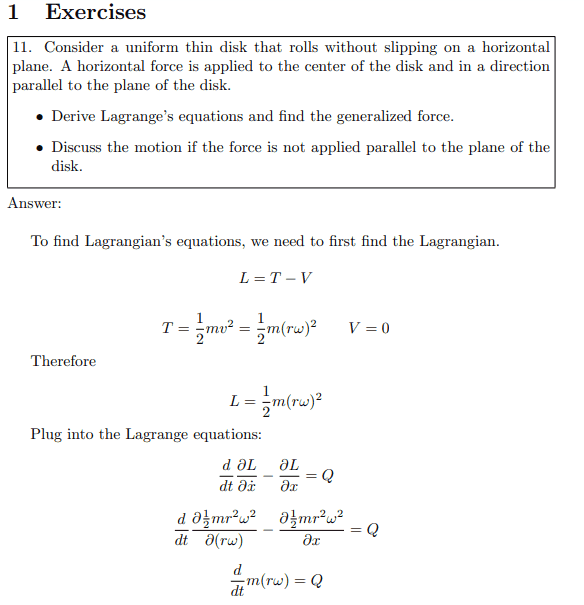# Problem about uniform thin disk

• I
AnotherWhy do they only think of kinetic energy of motion?
Why don't they think of both kinetic of motion and kinetic of rolling energy?

So. i think
## L = \frac{1}{2}mv^2 + \frac{1}{2}I \omega^2##
## L = \frac{1}{2}m(r \omega)^2 + \frac{1}{2}(\frac{1}{2}mr^2) \omega^2##
## L = \frac{3}{4} mr^2 \omega^2##

•etotheipi

•